Author: Tim Cummings

# Python in Minecraft 9 – railway track

To add railway track we need to have some normal rail and some powered rail. Next to every section of powered rail we need to provide a redstone torch.

First we define the items which are not defined in mcpi.block yet

```    RAIL = block.Block(66)
RAIL_POWERED = block.Block(27)
TORCH_REDSTONE = block.Block(76)
```

We are already placing a plain torch every 4 blocks. Now we want to place a redstone torch every 4 blocks but offset by 2. Next to the redstone torch we want two pieces of powered rail, at positions 1 and 2. The rest of the rail is normal rail. This code goes in the second loop where the full tunnel profile is created.

```    if x % 4 == 2:
mc.setBlock(x,y+1,z+1,TORCH_REDSTONE.id,5)
if x % 4 == 1 or x % 4 == 2:
mc.setBlock(x,y+1,z,RAIL_POWERED)
else:
mc.setBlock(x,y+1,z,RAIL)
```

Here is a screenshot showing the railway track in the tunnel.Here is the full code.

```import mcpi.minecraft as minecraft
import mcpi.block as block
mc=minecraft.Minecraft.create()
XMIN = -400 # x value at one end of tunnel.
XMAX = -200 # x value at other end of tunnel. Must be greater than XMIN
GROUND = 72 # y position of tunnel at each end
FLOOR = 10  # minimum value of y at bottom of tunnel
ZPOS = -244 # z position for full length of tunnel
TAIL = 10   # length of horizontal at each end of tunnel

# Define blocks currently missing from mcpi.block
STAIRS_STONE = block.Block(109)
RAIL = block.Block(66)
RAIL_POWERED = block.Block(27)
TORCH_REDSTONE = block.Block(76)

# constants for tunnelvertical function
kx = (XMAX + XMIN) / 2
ky = GROUND + TAIL - (XMAX - XMIN) / 2

# tunnelvertical returns a vertical position of the tunnel
# for each value of the x position (eastposition)
def tunnelvertical(eastposition):
y = abs(eastposition - kx) + ky
if y < FLOOR:
return FLOOR
if y > GROUND:
return GROUND
return y

# set the z coordinate for the tunnel which doesn't change for the full length
z = ZPOS

# Initial loop to create a route made of solid glass with a stone base
for x in range(XMIN,XMAX+1):
y = tunnelvertical(x)
mc.setBlocks(x,y,z-2,x,y+6,z+2,block.GLASS)
mc.setBlocks(x,y,z-1,x,y,z+1,block.STONE)

# Initialise previous value of y so can determine if stairs going up or down
yprev = tunnelvertical(XMIN)
# Second loop to convert solid glass into a tunnel
for x in range(XMIN+1,XMAX):
y = tunnelvertical(x)
# replace centre glass with air to make it a tunnel
mc.setBlocks(x,y+1,z-1,x,y+5,z+1,block.AIR)
# place a torch every 4 positions to light the tunnel
# variation 5 = torch facing up
# every 4 blocks
# pos 0: rail and normal torch
# pos 1: powered rail
# pos 2: powered rail and redstone torch
# pos 3: rail
if x % 4 == 0:
mc.setBlock(x,y+1,z+1,block.TORCH.id,5)
if x % 4 == 2:
mc.setBlock(x,y+1,z+1,TORCH_REDSTONE.id,5)
if x % 4 == 1 or x % 4 == 2:
mc.setBlock(x,y+1,z,RAIL_POWERED)
else:
mc.setBlock(x,y+1,z,RAIL)
# check if stairs are going up or down
if y > yprev:
# variation 0 = stairs ascending to the east
mc.setBlock(x,y,z-1,STAIRS_STONE.id,0)
if y < yprev:
# variation 1 = stairs ascending to the west
mc.setBlock(x-1,yprev,z-1,STAIRS_STONE.id,1)
yprev=y
```
Categories: CoderDojo

# Python in Minecraft 8 – stairs

To add stairs we need to know if the tunnel is descending or ascending to the east. We do this by creating a variable called yprev which stores the previous vertical position of the tunnel to the current position. We are creating the tunnel by increasing the value of x which means we are heading east. Hence if the current vertical position is more than the previous vertical position, the stairs are ascending to the east. If the current vertical position is less than the previous vertical position, the stairs are ascending to the west (descending to the east). If they are the same then we are not ascending or descending so no stairs are needed. At the end of the loop we need to store the current value of y in yprev so next time through the loop we will know the previous value. Check the full code to see where we initialise yprev before the loop.

```    # check if stairs are going up or down
if y > yprev:
# variation 0 = stairs ascending to the east
mc.setBlock(x,y,z-1,STAIRS_STONE.id,0)
if y < yprev:
# variation 1 = stairs ascending to the west
mc.setBlock(x-1,yprev,z-1,STAIRS_STONE.id,1)
yprev=y
```

We have decided to use stone stairs which are not predefined in Block.py in the modded mcpi. Hence we define it manually here.

```    STAIRS_STONE = block.Block(109)
```

Here is a screenshot showing the ascending stairs at the end of the tunnel.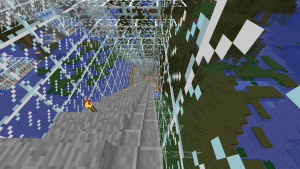Here is the full code.

```import mcpi.minecraft as minecraft
import mcpi.block as block
mc=minecraft.Minecraft.create()
XMIN = -400 # x value at one end of tunnel.
XMAX = -200 # x value at other end of tunnel. Must be greater than XMIN
GROUND = 72 # y position of tunnel at each end
FLOOR = 10  # minimum value of y at bottom of tunnel
ZPOS = -244 # z position for full length of tunnel
TAIL = 10   # length of horizontal at each end of tunnel

# Define blocks currently missing from mcpi.block
STAIRS_STONE = block.Block(109)

# constants for tunnelvertical function
kx = (XMAX + XMIN) / 2
ky = GROUND + TAIL - (XMAX - XMIN) / 2

# tunnelvertical returns a vertical position of the tunnel
# for each value of the x position (eastposition)
def tunnelvertical(eastposition):
y = abs(eastposition - kx) + ky
if y < FLOOR:
return FLOOR
if y > GROUND:
return GROUND
return y

# set the z coordinate for the tunnel which doesn't change for the full length
z = ZPOS

# Initial loop to create a route made of solid glass with a stone base
for x in range(XMIN,XMAX+1):
y = tunnelvertical(x)
mc.setBlocks(x,y,z-2,x,y+6,z+2,block.GLASS)
mc.setBlocks(x,y,z-1,x,y,z+1,block.STONE)

# Initialise previous value of y so can determine if stairs going up or down
yprev = tunnelvertical(XMIN)
# Second loop to convert solid glass into a tunnel
for x in range(XMIN+1,XMAX):
y = tunnelvertical(x)
# replace centre glass with air to make it a tunnel
mc.setBlocks(x,y+1,z-1,x,y+5,z+1,block.AIR)
# place a torch every 4 positions to light the tunnel
# variation 5 = torch facing up
if x % 4 == 0:
mc.setBlock(x,y+1,z+1,block.TORCH.id,5)
# check if stairs are going up or down
if y > yprev:
# variation 0 = stairs ascending to the east
mc.setBlock(x,y,z-1,STAIRS_STONE.id,0)
if y < yprev:
# variation 1 = stairs ascending to the west
mc.setBlock(x-1,yprev,z-1,STAIRS_STONE.id,1)
yprev=y
```
Categories: CoderDojo

# Python in Minecraft 7 – define tunnel route in function

In the previous example the tunnel was horizontal so the value of y for the tunnel was always constant. To make the tunnel route more complicated without having duplicate code we define a function called tunnelvertical(eastposition). For any value of the east position (x value) this function will return the vertical position of the tunnel.

```def tunnelvertical(eastposition):
y = abs(100 - (eastposition - XMIN))
if y < 10:
return 10
if y > 72:
return 72
return y
```

XMIN is a constant we have previously defined in the code showing where the tunnel starts. The shape of this tunnel is horizontal at ground level, then slopes down until it reaches level 10, goes horizontal for a while, then slopes up until it reaches ground level again and finishes off horizontal.

Here is the full code

```import mcpi.minecraft as minecraft
import mcpi.block as block
mc=minecraft.Minecraft.create()
XMIN = -400 # x value at one end of tunnel.
XMAX = -200 # x value at other end of tunnel. Must be greater than XMIN
GROUND = 72 # y position of tunnel at each end
FLOOR = 10  # minimum value of y at bottom of tunnel
ZPOS = -222 # z position for full length of tunnel
TAIL = 10   # length of horizontal at each end of tunnel

# constants for tunnelvertical function
kx = (XMAX + XMIN) / 2
ky = GROUND + TAIL - (XMAX - XMIN) / 2

# tunnelvertical returns a vertical position of the tunnel
# for each value of the x position (eastposition)
def tunnelvertical(eastposition):
y = abs(eastposition - kx) + ky
if y < FLOOR:
return FLOOR
if y > GROUND:
return GROUND
return y

# set the z coordinate for the tunnel which doesn't change for the full length
z = ZPOS

# Initial loop to create a route made of solid glass with a stone base
for x in range(XMIN,XMAX+1):
y = tunnelvertical(x)
mc.setBlocks(x,y,z-2,x,y+6,z+2,block.GLASS)
mc.setBlocks(x,y,z-1,x,y,z+1,block.STONE)

# Second loop to convert solid glass into a tunnel
for x in range(XMIN+1,XMAX):
y = tunnelvertical(x)
# replace centre glass with air to make it a tunnel
mc.setBlocks(x,y+1,z-1,x,y+5,z+1,block.AIR)
# place a torch every 4 positions to light the tunnel
if x % 4 == 0:
mc.setBlock(x,y+1,z+1,block.TORCH.id,5)
```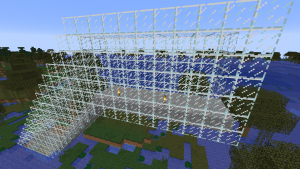Categories: CoderDojo

# Python in Minecraft 6 – stone floor tunnel with torches

In the previous example the tunnel was glass all around. To make a more interesting tunnel this example adds a stone floor and adds a torch. We will develop this tunnel in two stages. The first stage is to create a profile showing what a cross-section of the tunnel will look like for any value of x.

`nano tunnelprofile2.py`

```import mcpi.minecraft as minecraft
import mcpi.block as block
mc=minecraft.Minecraft.create()
x = -190
y = 72
z = -222
mc.setBlocks(x,y,z-2,x,y+6,z+2,block.GLASS)
mc.setBlocks(x,y,z-1,x,y,z+1,block.STONE)
mc.setBlocks(x,y+1,z-1,x,y+5,z+1,block.AIR)
mc.setBlock(x,y+1,z+1,block.TORCH.id,5)```

`python3 tunnelprofile2.py`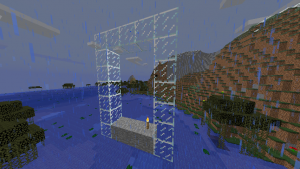The second stage is to create a loop to draw each cross section of the tunnel at each value of x between xmin and xmax. Note that at the ends we ant the wall of glass to stay so that lava and water don’t flow into the tunnel should it get built next to these liquids. We have achieved this with two loops. The first loop goes the full length of the tunnel from xmin to xmax. The second loop which adds air and torches starts and ends one position in from the ends. Torches are added every 4 positions by using the modulo (%) operator.

`nano stonefloortunnel.py`

```import mcpi.minecraft as minecraft
import mcpi.block as block
mc=minecraft.Minecraft.create()
xmin = -224
xmax = -200
y = 72
z = -222
for x in range(xmin,xmax+1):
mc.setBlocks(x,y,z-2,x,y+6,z+2,block.GLASS)
mc.setBlocks(x,y,z-1,x,y,z+1,block.STONE)
for x in range(xmin+1,xmax):
mc.setBlocks(x,y+1,z-1,x,y+5,z+1,block.AIR)
if x % 4 == 0:
mc.setBlock(x,y+1,z+1,block.TORCH.id,5)```

`python3 stonefloortunnel.py`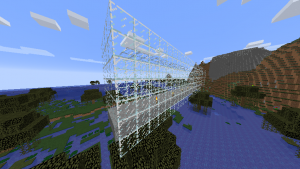Categories: CoderDojo

# Python in Minecraft 5 – hollow tunnel

## Creating a hollow tunnel with walls at each end

To create an air space in the tunnel we can use another loop. However, the second loop should not go all the way to the ends because we want walls at each end. This is to stop water and lava flowing into our tunnel should the ends of the tunnel meet these liquids.

`nano hollowglasstunnel.py`

```import mcpi.minecraft as minecraft
import mcpi.block as block
mc=minecraft.Minecraft.create()
xmin = -224
xmax = -200
y = 72
z = -222
for x in range(xmin,xmax+1):
mc.setBlocks(x,y,z-2,x,y+6,z+2,block.GLASS)
for x in range(xmin+1,xmax):
mc.setBlocks(x,y+1,z-1,x,y+5,z+1,block.AIR)
```

`python3 hollowglasstunnel.py`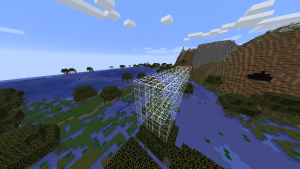Categories: CoderDojo